In this video we will be learning how to declare variables in word problems. After you finish this lesson, view all of our Algebra 1 lessons and practice problems.

## Example of Declaring Variables in Algebra

The length of a rectangle is 5 units longer than the width. If the perimeter is 22 units, how long is the width?

Let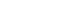$w = width$

Let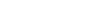$w + 5 = length$

Perimeter Formula: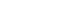$P=2l+2w$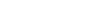$w + w + w + 5 + w + 5 = 22$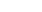$w = 3$

## Examples of Declaring Variables

### Example 1

Linda spent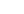$\38$ for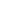$3$ notebooks; a box of pencils at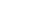$\1.50$ and a box of pens at$\1.70$. What is the price of each notebook?

First, declare variables

Let$n$= cost of each notebook

Next, use sentence to create equation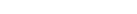$Total= 3n + 1.50+1.70$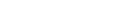$38= 3n + 1.50 + 1.70$

Now, we can solve for$n$

Add the constants on the right side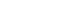$38=3n+3.2$

Then subtract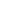$3.2$ to both sides of the equation.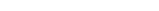$38-3.2=3n+3.2-3.2$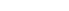$34.8=3n$

To solve$n$, let’s divide both sides by$3$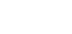$\dfrac{34.8}{3}=\dfrac{3n}{3}$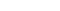$11.6=n$

Therefore, the cost of each notebook is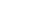$\11.6$

### Example 2

Krishna bought a total of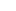$37$ pens. She bought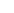$11$ more boxes of colored pink pens than of colored red pens. How many of each did she buy?

First, declare variables

Let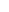$p$= for colored pink pens

Let$r$= colored red pens

Next, use sentence to create equation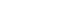$Total=p +r$

Since p has$11$ more pens than$r$, therefore: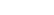$p=r+11$

Substitute the values of$p$ to the equation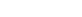$37=(r+11) + r$

Now, we can solve for$r$$37=(r+11) + r$

3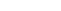$7=2r+11$

Then subtract$11$ to both sides of the equation.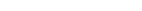$37-11=2r+11-11$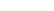$26=2r$

To solve$r$, let’s divide both sides by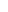$2$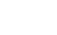$\dfrac{26}{2}=\dfrac{2r}{2}$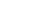$13=r$

Therefore, there are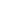$13$ colored red pens.

Let’s go back to the problem and substitute the value of$p$.$p=r+11$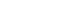$p=13+11$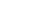$p=24$

## Video-Lesson Transcript

Let’s go over declaring variables.

When we have word problems, we may start writing down equations based on the given then try to solve it.

But if we lost track of what the variables are, it’s highly possible to get an incorrect answer.

Normally, declaring variables is like this:

Let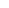$x$ equal a number of something.

Or let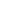$y$ equal the width of a shape.

Let’s have an example.

A book store charges$\20$ for the first book and$\15$ for each additional book. Write an expression to represent the total cost of buying any number of books.

Let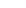$x =$ number of books.

Now we need to know the total cost based on the information given.

Now, we have

cost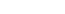$= \20 + \15$ times number of additional books

Let’s use$x$ in this equation.

cost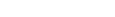$= \20 + \15 (x - 1)$$x$ is the number of additional books. So we subtract the first book from cost$x$.

For example:

We bought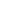$10$ books. The cost is going to be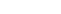$20 + 15 \times9$ additional.

It’s not$10$ because the first book is valued at$\20$.Let’s take a look at another example.

The length of a rectangle is$5$ units longer than the width. If the perimeter is$22$ units, how long is the width?

Let$w = width$

Let$w + 5 = length$

Now, let’s draw a rectangle.

Label the two as “w” for width and label the length as “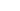$w + 5$“.

The perimeter is$w + w + w + 5 + w + 5 = 22$

Since we already labeled the rectangle, it’ll be easier.

Let’s continue solving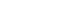$4w + 10 = 22$

Then subtract$10$ to both sides of the equation.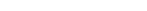$4w + 10 - 10 = 22 - 10$

This becomes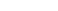$4w = 12$

To solve “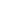$w$“, let’s divide both sides by$4$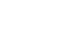$\dfrac{4w}{4} = \dfrac{12}{4}$$w = 3$

Now, let’s go back to the problem and substitute the value of$w$.

If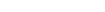$w = width = 3$,

then the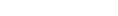$w + 5 = length = 8$.